Function Repository Resource:

# MultisetSymmetricDifference

Get the symmetric difference of the given lists

Contributed by: George Beck
 ResourceFunction["MultisetSymmetricDifference"][L1,L2,…] gives the list of elements that occur an odd number of times in the lists L1,L2,….

## Details and Options

For two lists with no duplicates, ResourceFunction["MultisetSymmetricDifferences"] gives the usual symmetric difference of the lists considered as sets, which is the Complement of the Union and Intersection.
The result is sorted.

## Examples

### Basic Examples (3)

Here is the symmetric difference of two sets:

 In:=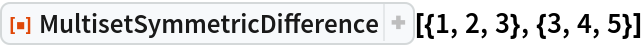Out=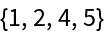Another example:

 In:=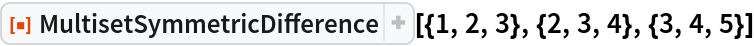Out=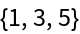Like Union, Intersection, and Complement, a single list is pruned of duplicates and sorted:

 In:=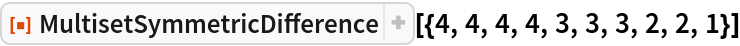Out=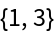### Possible Issues (4)

Define two lists, one with a duplicated element:

 In:=The lists are equal up to duplicates, so the set symmetric difference is empty:

 In:=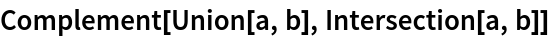Out=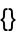The element 1 occurs an odd number of times in the multiset sum of the lists:

 In:=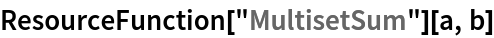Out=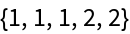Therefore, 1 is in the multiset difference:

 In:=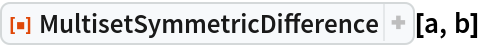Out=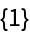George Beck

## Requirements

Wolfram Language 11.3 (March 2018) or above

## Version History

• 1.0.0 – 10 May 2019